Multilevel Thresholding

Multilevel thresholding is useful to extract several kinds of objects whose pixel values are not close.

I implemented automatic multilevel thresholding function (ThresholdML) by following .

You can use the function as shown below.

library(devtools)
install_github("ShotaOchi/imagerExtra")
library(imagerExtra)
a <- crop.borders(a, 1000, 500) %>% resize(., -20, -20, interpolation_type = 5L)
g <- G(a)
b <- ThresholdML(g, 4)
layout(matrix(1:2, 1, 2))
plot(g, main = "Original")
plot(b, main = "Multilevel")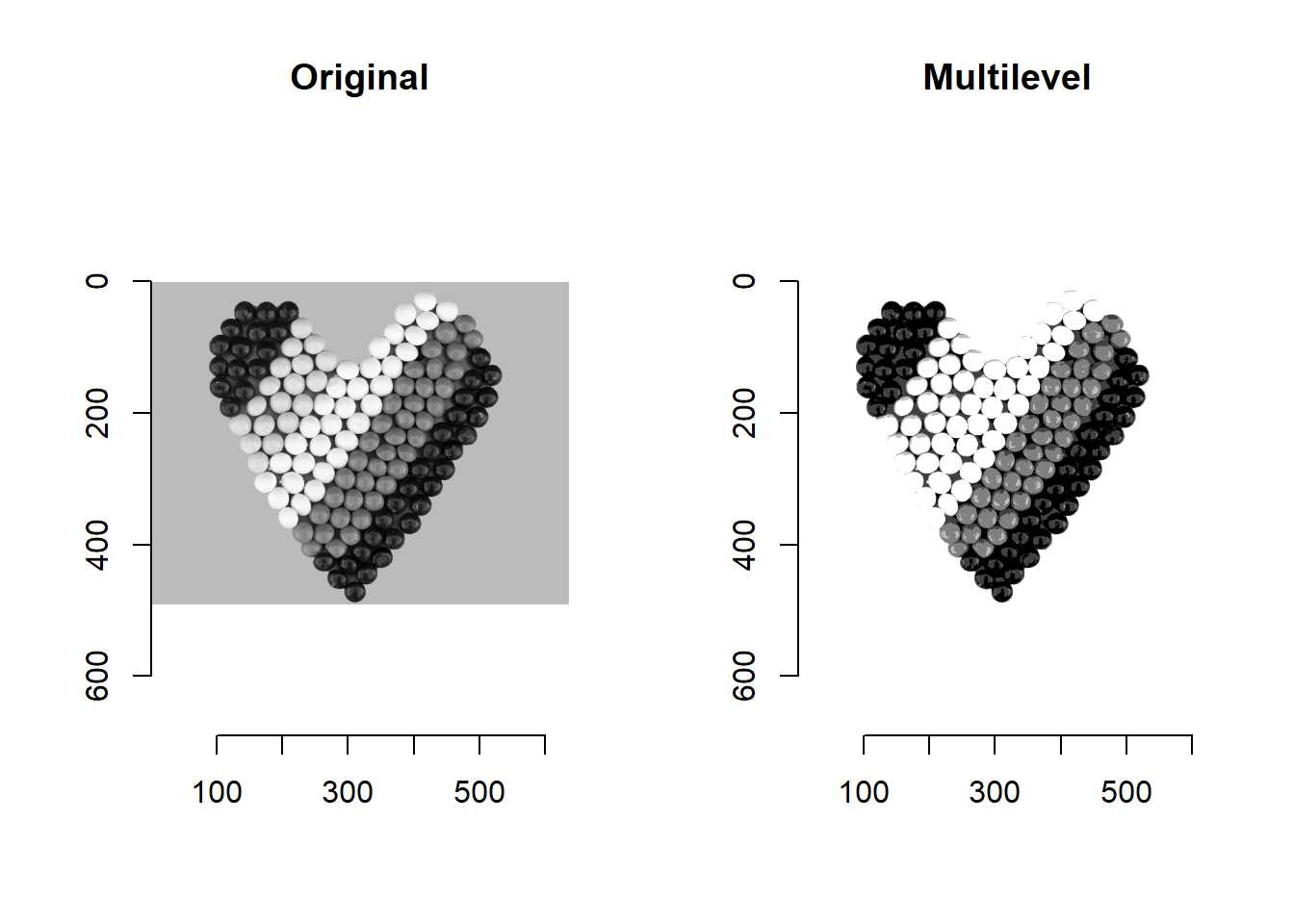We can see three kinds of balls were extracted.

There are four kinds of balls in the original image, but I don’t mind.

The background is annoying. We can remove it.

bg <- bucketfill(a, 1, 1, color="black", sigma = .1) %>% grayscale()
bg <- bg != 0
s <- (b + 1) * bg
plot(s)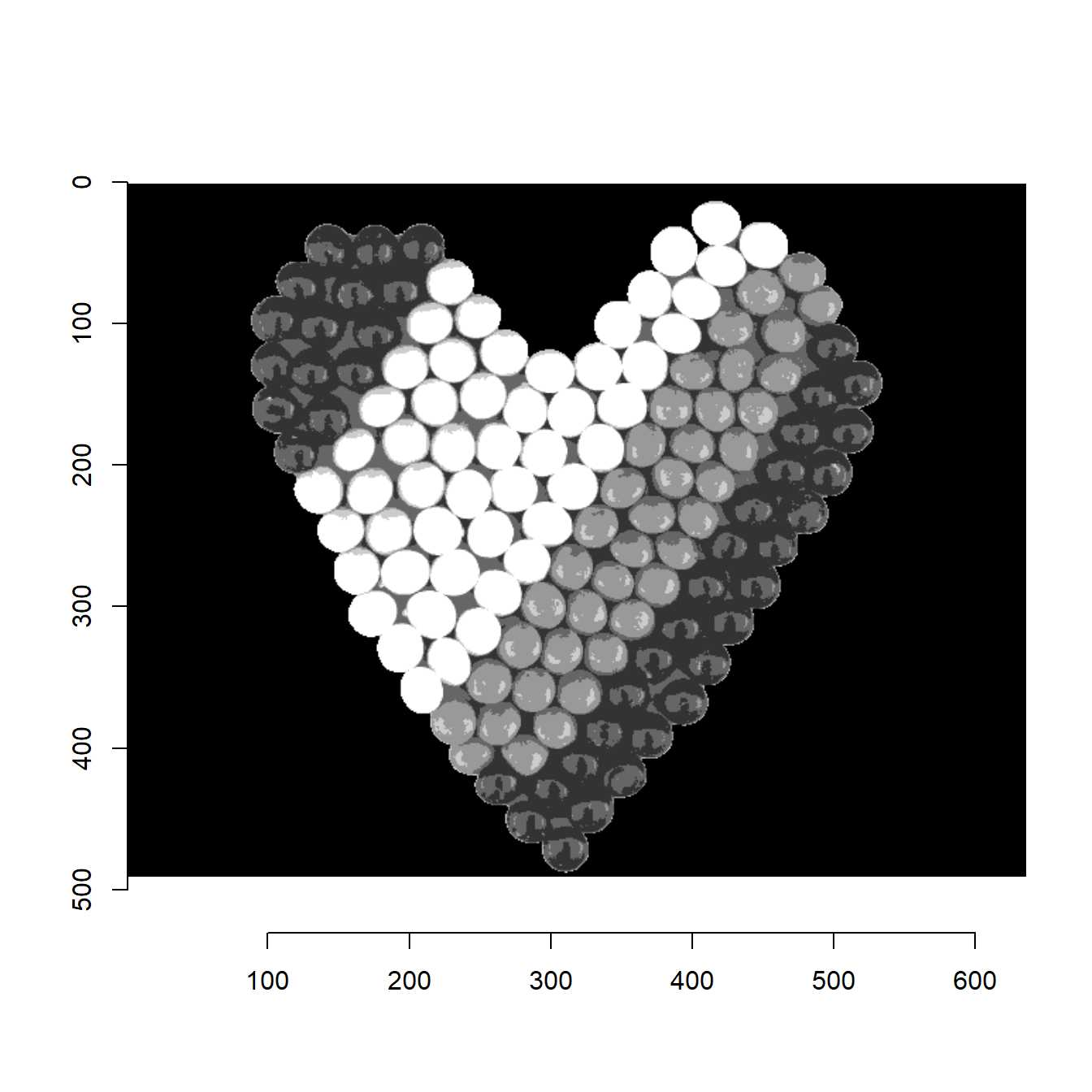Note that ThresholdML uses an artificial bee colony algorithm.

That’s why ThresholdML may return different result each time.

gg <- boats %>% grayscale()
test1 <- sapply(1:1000, function(x) ThresholdML(gg, 4, returnvalue = TRUE)) %>% t()
boxplot(test1, main = "fast", xlab = "thresholds", ylab = "threshold value")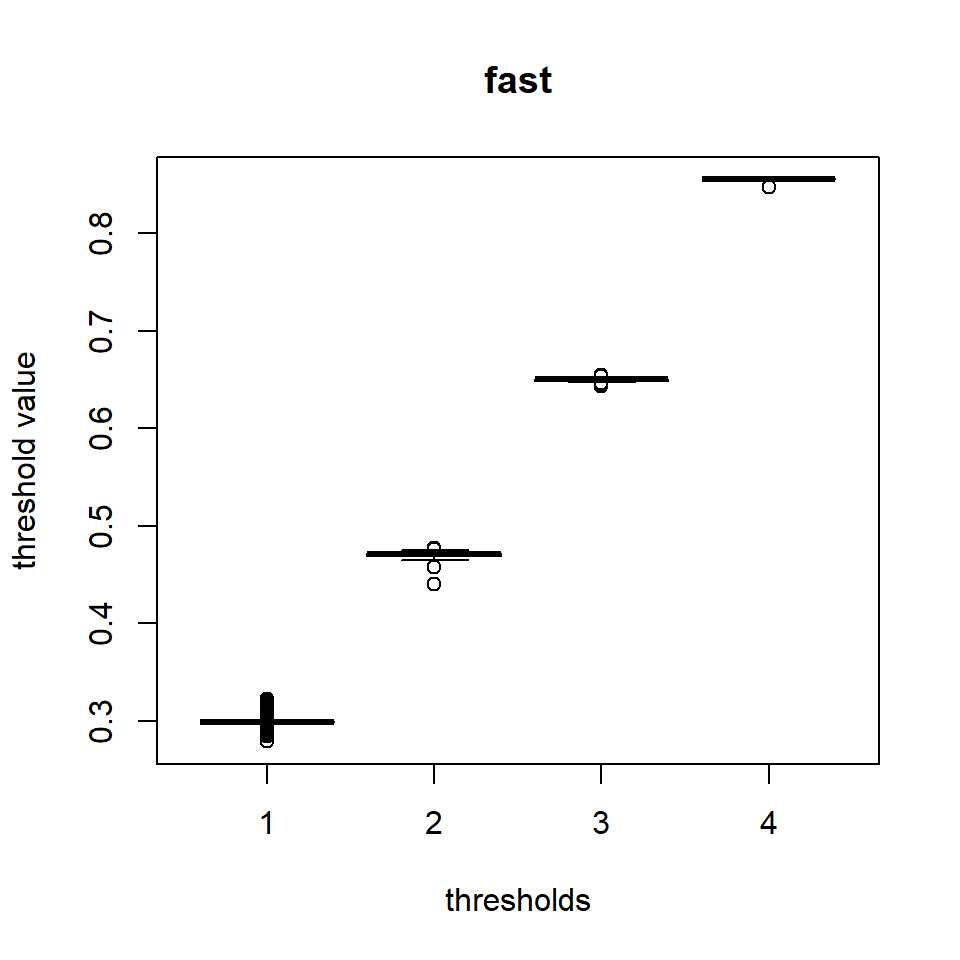We have two options to reduce the variance of the threshold values.

One is to set thr as “precise”.

Another is to set thr as “manual” and tune the parameters.

test2 <- sapply(1:1000, function(x) ThresholdML(gg, 4, thr = "precise", returnvalue = TRUE)) %>% t()
boxplot(test2, main = "precise", xlab = "thresholds", ylab = "threshold value")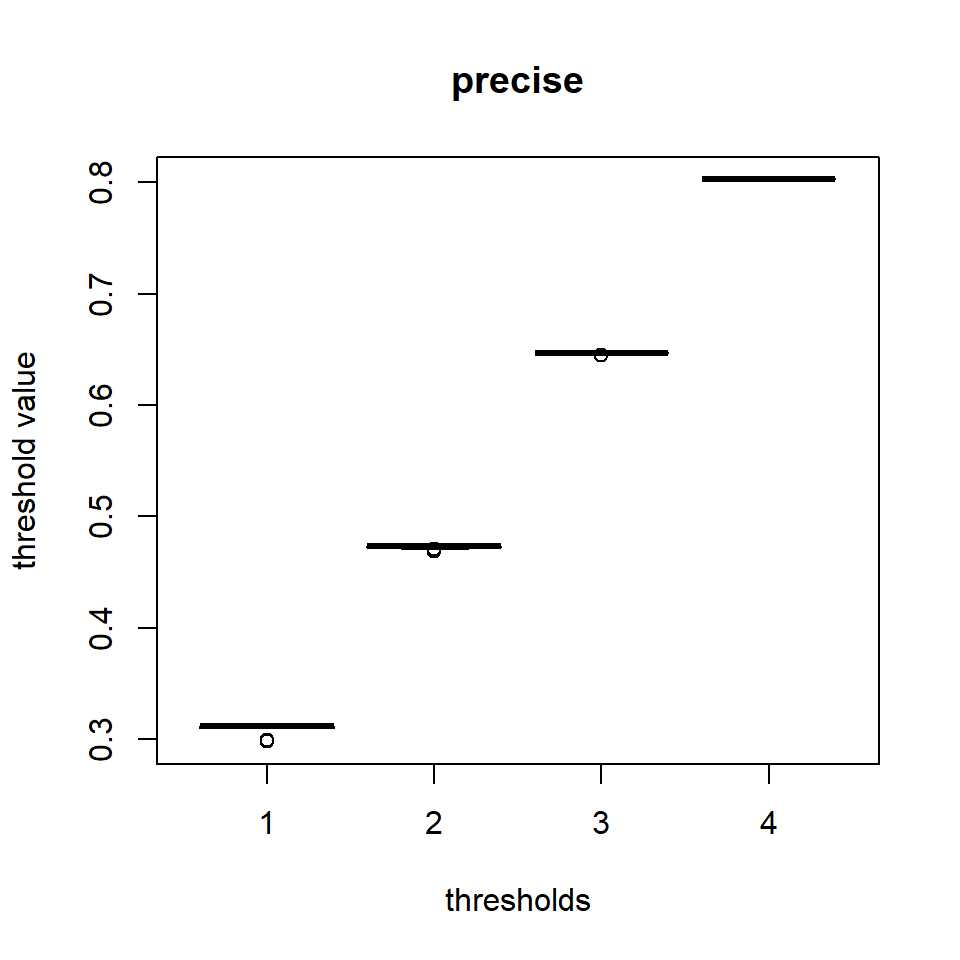test3 <- sapply(1:1000, function(x) ThresholdML(gg, 4, thr = "manual", sn = 200, mcn=200, limit = 10, intervalnumber = 2000, returnvalue = TRUE)) %>% t()
boxplot(test3, main = "manual", xlab = "thresholds", ylab = "threshold value")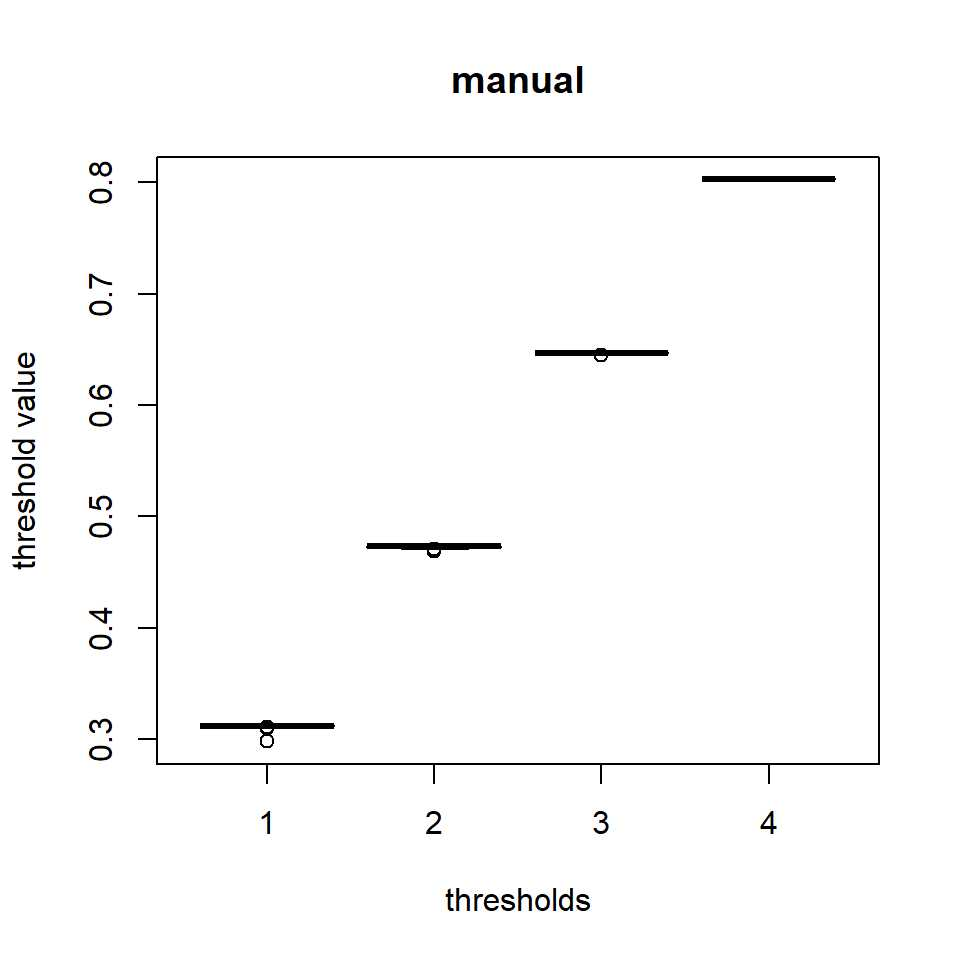You can specify threshold values by setting thr to a numeric vector as shown below.

thr <- c(0.15, 0.3, 0.45, 0.6)
m <- ThresholdML(g, thr = thr)
plot(m)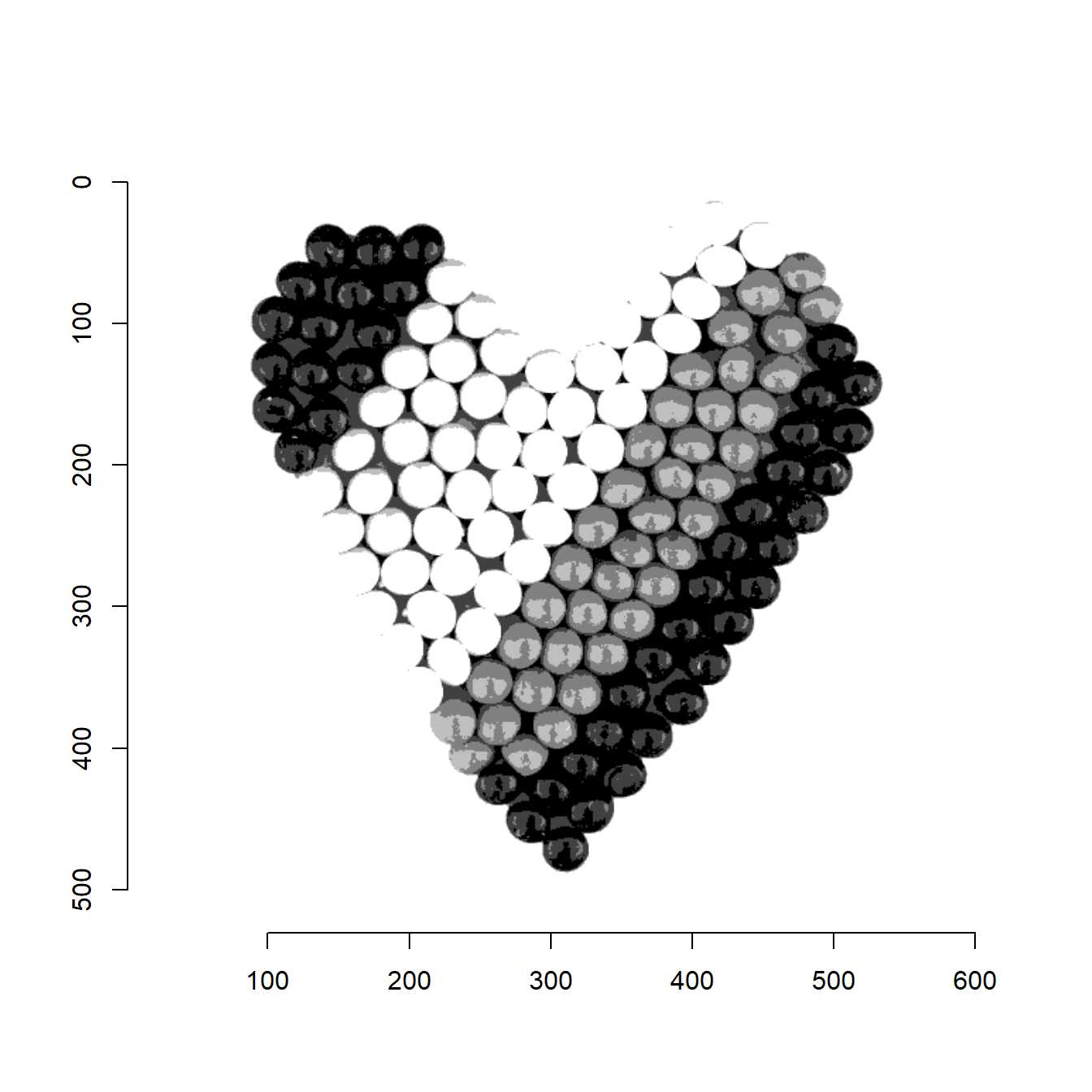Ming-HuwiHorng (2011). Multilevel thresholding selection based on the artificial bee colony algorithm for image segmentation. Expert Systems with Applications.